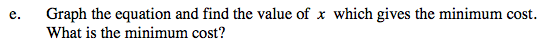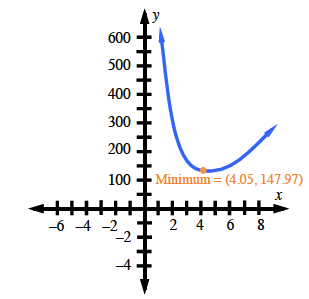### Home > PC > Chapter 9 > Lesson 9.1.3 > Problem9-39

9-39.
1. The manufacturing company you work for has been hired to produce a 100 cubic foot box. It must have a square base and no top. The material for the base costs $3 per square foot and the material for the sides costs$1 per square foot. We want to write an equation for the cost of the box as a function of x, the length of one edge of the base. Homework Help ✎

1. Write an expression for the cost of the base in terms of x.

2. Express the cost of the sides in terms of x and h, the height of the box.

3. Express the total cost of the box in terms of x and h.

4. Use the fact that the volume of the box is 100 cubic feet to eliminate h from the equation you just wrote and express the cost solely in terms of x.

5. Graph the equation and find the value of x which gives the minimum cost. What is the minimum cost?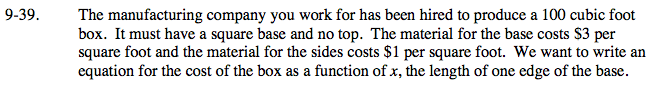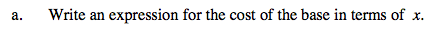Write and expression for the area and multiply that by the cost per square foot.

Area: x2
Cost: 3x2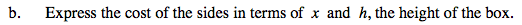Write an expression for the area of one side.
Write an expression for the area of four sides.
Write an expression for the cost of four sides.

Area: 4xh
Cost: (1)(4xh) = 4xh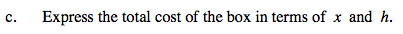This is the sum of your expressions from parts (a) and (b).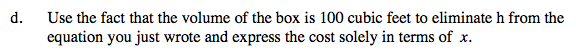100 = x2h

$h = \frac{100}{x^2}$

Substitute 'h' into the equation in part (c).